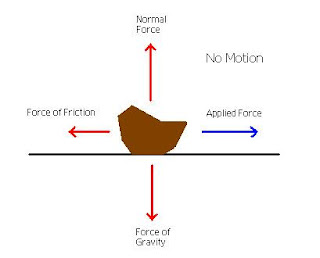## Translate

### (adsbygoogle = window.adsbygoogle || []).push({}); Friction :

Friction is the force that opposes the motion of an object moving relative to another.

(OR)
A force acting in the opposite direction to the motion of the body is called “force of friction or simply friction”. It is of the following two types :

###1. Static friction .

The friction, experienced by a between two body's that aren't moving relative to each other, when at rest, is known as “static friction”while kinetic friction acts between objects in motion.

### 2. Dynamic friction.

When the body is in moving condition the friction force occurred by the body is know as Dynamic Friction.

#### (a) Slide friction andThe friction, experienced by a body, when it slides over another body, is known as “sliding friction”. The friction experienced by a body, when balls or rollers are interposed between the two surfaces, is known as “rolling friction”.

#### Laws of Static Friction :

1. The force of friction always acts in a direction, opposite to that which the body tends to move.
2. The magnitude of force of friction is exactly equal to the force, which tends the body to move.
3. The force of friction is independent of the area of contact between the two surfaces.
4. The force of friction depends upon the roughness of the surfaces.

#### Laws of Dynamic or Kinetic Friction :

Following are the laws of dynamic or kinetic friction :
1. The force of friction always acts in a direction, opposite to that in which the body tends to move.
2. The magnitude of the kinetic friction bears a constant ratio to the normal reaction between the two surfaces.
3. For moderate speeds, the force of friction remains constant. But it decreases slightly with the increase of speed.

#### What isLimiting Friction ?

The maximum value of friction force, which comes into play, when a body just begins to slide over the surface of the other body, is known as “Limiting friction”.

#### Coefficient of Friction :

It is defined as the ratio limiting friction(F) to the normal reaction(RN) b/w the two bodies. It is generally denoted by u. Mathematically, Coefficient of friction, u= F/RN